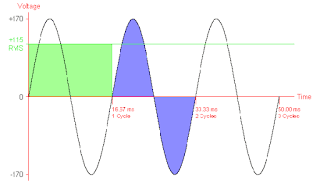### 60w and 100w bulb connected in series which glow brighter?

1. The rated power on two bulbs 60w and 100w is for parallel connection from the manufacturer only mean when u connect both in parallel then both used the same amount of power as rated.
2. if 60w and 100w bulb connected in series then both bulbs dissipate the different amounts of power compared to rated power.
3. remember when load connected in series current will be the same for all and if connected in parallel voltage will the same for all.

#### How? Calculation:-

Let 220 volt is the supply in parallel(house supply)
Because both connected in series then, resistance
=R(total)=R(60w)+R(100w),
For further calculation, we need to know the resistance of both bulb,
Because rated bulb 60w and 100w is for only parallel connection, P(power)=V(parallel)²/R(bulb)
=R(60w)=(220)²/60=806 ohm,
=R(100w)=(220)²/100=484 ohm.
Then for series connection:-
R(total)=(806+484) ohm=1290 ohm
So V(total)=I*R(total),
200=I*1290
I=0.17 amp,

#### further:-

Current flowing in circuit is 0.17 amp Power by 200v. Because the power in series is different from rated power.
Then power consumed by 60w rated bulb in series is
=P(60w)=I²*R(60w)
=P(60w)=(0.17)²*806
=P(60w)=23.29 watt
And power consumed by 100w rated bulb in series is
=P(100w)=I²*R(100w)
=P(100w)=(0.17)²*484
=P(100w)=13.98 watt.

#### conclusion:- if 60w and 100w rated bulb connected in series then both consume power as 23.29w and 13.98 w respectively then 60w rated bulb more consume power then 100w rated bulb."Because greater resistance in series consumes high power and greater resistance in parallel consume low power".So, 60w will glow brighter than 100w in series connection.

Capacitor workCredit:http://www.co-bw.com/

The nature of reality is that it does not store of charge. how?
Not inside the dielectric material because dielectric material is actually an insulator.

• Let you connected a capacitor in the dc circuit in series. So when positive current flow the first plate(conductor) of capacitor charge, but not flow through capacitor because insulator material inside it. Ultimately dc current not flow or block by the capacitor.
• let connected a capacitor in ac circuit in Series, so when a positive half cycle of ac flow capacitor's first conductive plate then it will charge and when negative half cycle of ac flow the second conductive plate charge as well as first plate discharge. and then positive half cycle flows first plate charge and second plate discharge.
• current never flows inside a capacitor because of insulation (like ceramic, mica, air, etc.)
Is RMS is DCCredit:https://www.quora.com/

• Every waveform RMS value is the DC-equivalent value.
• its a way of expression, actually its RMS voltage/current calculation is a dc voltage/current value. And frequency is not considered in RMS value.
• An voltage/current RMS value is written everywhere not peak value.
• example:- 220v RMS house wiring or 335v peak ac. 110v RMS value or 154v peak ac.
• more examples:- if RMS value is 10v AC its means you can deliver the same amount of power via dc source 10v DC
• but the nature of reality is that AC is oscillating and dc is constant theoretically.

If it carry 30apm dc it also carry 30apm ac(RMS).